# How to make Optional Parameters in C#

## How to make Optional Parameters in C# with Examples

In this article, I am going to discuss How to make Optional Parameters in C# with some examples. This is also one of the most frequently asked interview questions. So here we will discuss the different options that are available in C# to make the method parameters as optional.

We can make the method parameters as optional in C# in many different ways as follows.

1. Using parameter array
3. Specify parameter defaults
4. Using OptionalAttribute

So, let us discuss all these options one by one

##### Using parameter array

Let’s us discuss how to make method parameters as optional using parameter array with an example.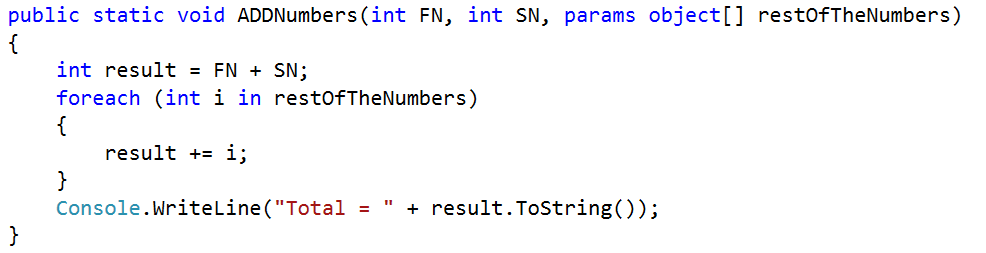The above ADDNumbers function allows the user to add 2 or more numbers. The FN and SN parameters are mandatory parameters whereas the restOfTheNumbers parameter is optional.

If the user wants to add just two numbers, then he can invoke the method as shown below

On the other hand, if the user wants to add 5 numbers, then he can invoke the method as shown below.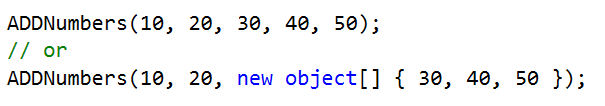The parameter array must be the last parameter in the formal parameter list. The following function will not be compiled as the parameter array not the last parameter of the parameter list.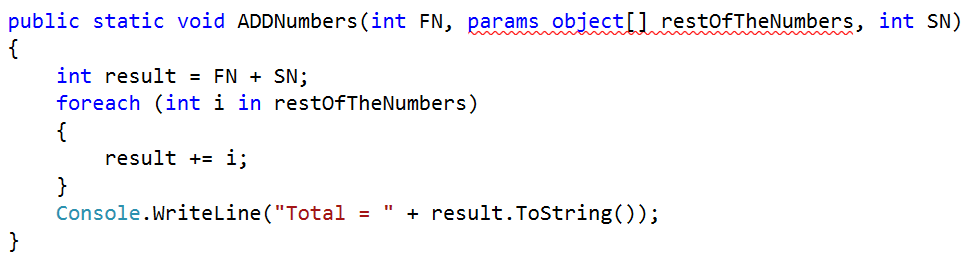The complete example is given below.

```using System;
namespace OptionalParameter
{
class Program
{
static void Main(string[] args)
{
ADDNumbers(10, 20, new object[] { 30, 40, 50 });
}

public static void ADDNumbers(int FN, int SN, params object[] restOfTheNumbers)
{
int result = FN + SN;
foreach (int i in restOfTheNumbers)
{
result += i;
}
Console.WriteLine("Total = " + result.ToString());
}
}
}```

OUTPUT: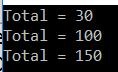Let us discuss how to make method parameters optional using method overloading. Let create the following method which will add any number of integers.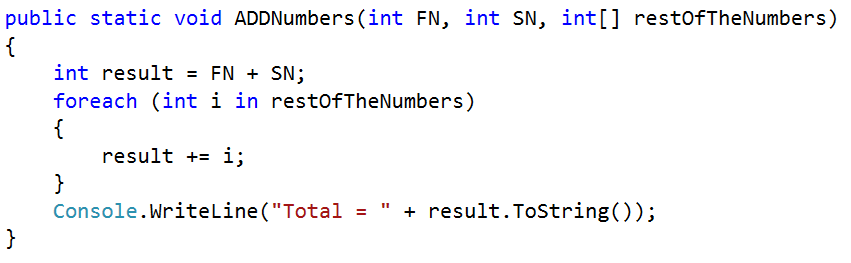If you want to add 5 integers – 10, 20, 30, 40 and 50 then you need to call the method as shown below.At the moment all the 3 method parameters are mandatory. Now, if I want to add just 2 numbers, then I can invoke the method as shown below.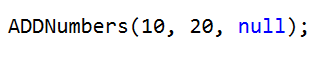Notice that, here I am passing null as the argument for the 3rd parameter. We can make the 3rd parameter as optional by overloading ADDNumbers() function which takes two parameters as shown below.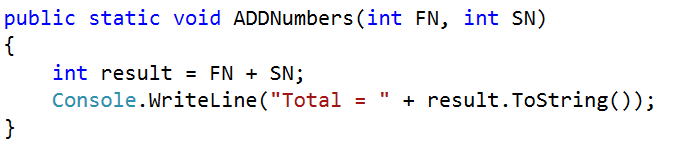Now, we have 2 overloaded versions of ADDNumbers() function. If we want to add just 2 numbers, then I can use the overloaded version of ADDNumbers() function which takes 2 parameters as shown below.

Similarly, if I want to add 3 or more numbers, then I can use the overloaded version of ADDNumbers() function which takes 3 parameters as shown below.

ADDNumbers(10, 20, new int[] { 30, 40 });

The complete example is given below

```using System;
namespace OptionalParameter
{
class Program
{
static void Main(string[] args)
{
ADDNumbers(10, 20, new int[] { 30, 40, 50 });

}

public static void ADDNumbers(int FN, int SN, int[] restOfTheNumbers)
{
int result = FN + SN;
foreach (int i in restOfTheNumbers)
{
result += i;
}
Console.WriteLine("Total = " + result.ToString());
}

public static void ADDNumbers(int FN, int SN)
{
int result = FN + SN;
Console.WriteLine("Total = " + result.ToString());
}
}
}```

OUTPUT: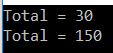##### Specify parameter defaults

Let us discuss how to specify the parameter defaults to make the make method parameters optional. We can make the method parameter optional by specifying a default value of null as shown below.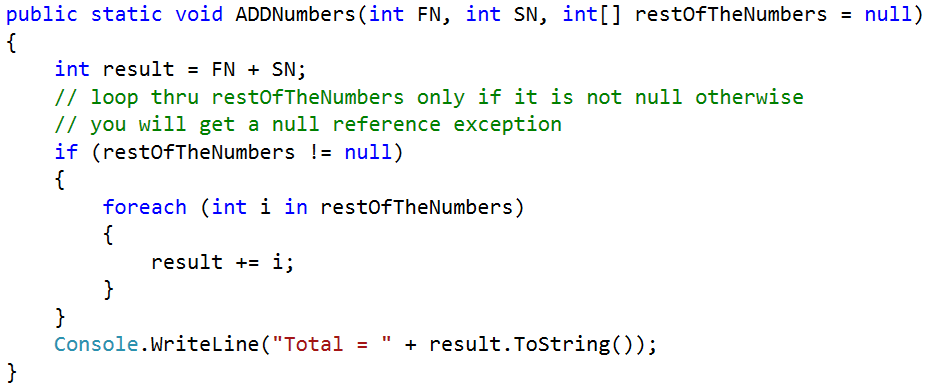As we have specified a default value for the 3rd parameter now it becomes optional. So, if we want to add just 2 numbers, we can invoke the function as shown below.

The Optional parameters in C# must appear after all the required parameters. The following method will not be compiled. This is because, we are making parameter “restOfTheNumbers” optional, but it appears before the required parameters “SN”.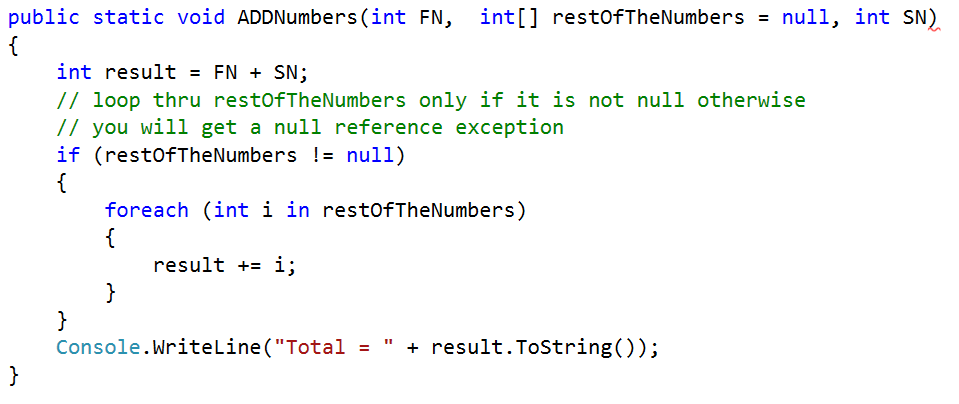###### Named Parameters:

In the following method, the parameters “b” & “c” are optional.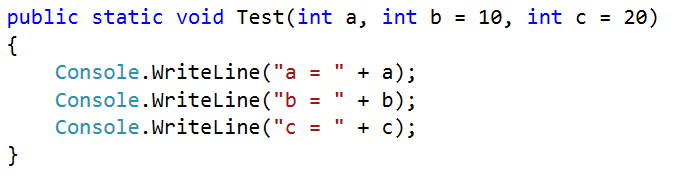When we invoke the above method as shown below “1” is passed as the argument for parameter “a” and “2” is passed as the argument for parameter “b” by default.

Test(1, 2);

My intention is to pass “2” as the argument for parameter “c”. To achieve this we can make use of named parameters, as shown below. Notice that, I have specified the name of the parameter for which value “2” is being passed.

Test(1, c: 2);

The complete example is given below.

```using System;
namespace OptionalParameter
{
class Program
{
static void Main(string[] args)
{
ADDNumbers(10, 20, new int[] { 30, 40, 50 });
Test(1, 2);
Test(1, c:2);
}

public static void ADDNumbers(int FN, int SN,  int[] restOfTheNumbers = null)
{
int result = FN + SN;
// loop thru restOfTheNumbers only if it is not null otherwise
// you will get a null reference exception
if (restOfTheNumbers != null)
{
foreach (int i in restOfTheNumbers)
{
result += i;
}
}
Console.WriteLine("Total = " + result.ToString());
}

public static void Test(int a, int b = 10, int c = 20)
{
Console.WriteLine("a = " + a);
Console.WriteLine("b = " + b);
Console.WriteLine("c = " + c);
}
}
}```

OUTPUT: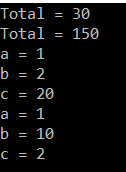##### Using OptionalAttribute

Let us now discuss how to make method parameters as optional by using the OptionalAttribute that is present in System.Runtime.InteropServices namespace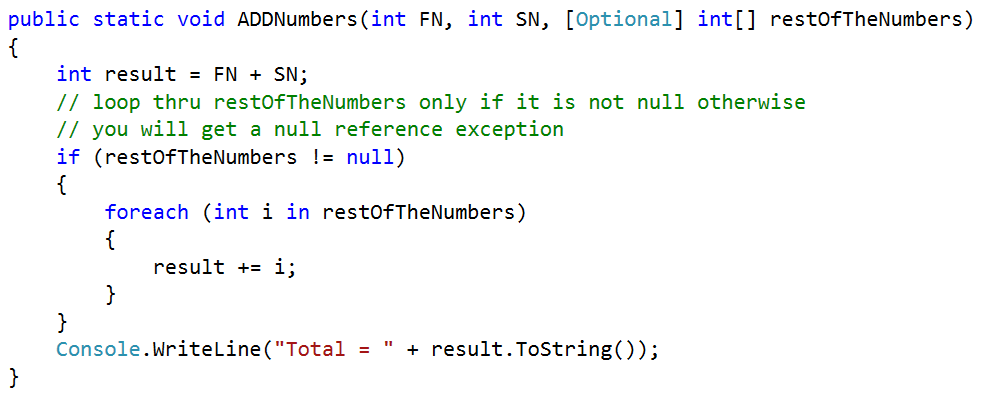Here we are making the restOfTheNumbers as optional by using the [Optional] attribute. The complete example is given below.

```using System;
using System.Runtime.InteropServices;

namespace OptionalParameter
{
class Program
{
static void Main(string[] args)
{
ADDNumbers(10, 20, new int[] { 30, 40, 50 });

}

public static void ADDNumbers(int FN, int SN, [Optional] int[] restOfTheNumbers)
{
int result = FN + SN;
// loop thru restOfTheNumbers only if it is not null otherwise
// you will get a null reference exception
if (restOfTheNumbers != null)
{
foreach (int i in restOfTheNumbers)
{
result += i;
}
}
Console.WriteLine("Total = " + result.ToString());
}
}
}```

OUTPUT: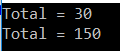In the next article, I will discuss how to create and use Indexers in C#. Here, in this article, I try to explain how to make Optional Parameters in C# with some examples.